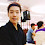## 11/08/2011

### 8 point algorithm (Matlab source code) / The method to get the Fundamental Matrix and the Essential matrix

Created Date : 2011.8
Language : Matlab
Tool : Matlab 2010
Library & Utilized : -
Reference : Multiple View Geometry (Hartly and Zisserman)
etc. : Intrinsic Parameter, 2 adjacent images, matching points

This code is 8 point algorithm.
If we know over 8 corresponding points between two images, we can know Rotation and Translation of camera movement using 8 point algorithm.
The 8 point algorithm is well known in the vision major field.
The algorihtm is introduced at the Multiple View Geometry Book and many websites.

Have you ever listened Fundamental matrix song? The song is very cheerful. ^^

My code is very simple. so I believe my code will be useful to you.
I will upload RANSAC version later.
Thank you.

github url :https://github.com/MareArts/8point-algorithm
(I used 'getCorrectCameraMatrix' function of Isaac Esteban author.)

main M code..
```%//////////////////////////////////////////////////////////////////////////
%// Made by J.H.KIM, 2011 / feelmare@daum.net, feelmare@gmail.com        //
%// blog : http://feelmare.blogspot.com                                  //
%// Eight-Point Algorithm
%//////////////////////////////////////////////////////////////////////////

clc; clear all; close all;

% Corresponding points between two images
% sample #1 I11.jpg, I22.jpg
%{
m1 = I11; m2 = I22;
%}

%sample #2 I1.jpg, I2.jpg
m1 = I1; m2 = I2;

s = length(m1);
m1=[m1(:,1) m1(:,2) ones(s,1)];
m2=[m2(:,1) m2(:,2) ones(s,1)];
Width = 800; %image width
Height = 600; %image height

% Intrinsic Matrix
K = intrinsic_matrix;

% The matrix for normalization(Centroid)
N=[2/Width 0 -1;
0 2/Height -1;
0   0   1];

%%
% Data Centroid
x1=N*m1'; x2=N*m2';
x1=[x1(1,:)' x1(2,:)'];
x2=[x2(1,:)' x2(2,:)'];

% Af=0
A=[x1(:,1).*x2(:,1) x1(:,2).*x2(:,1) x2(:,1) x1(:,1).*x2(:,2) x1(:,2).*x2(:,2) x2(:,2) x1(:,1) x1(:,2), ones(s,1)];

% Get F matrix
[U D V] = svd(A);
F=reshape(V(:,9), 3, 3)';
% make rank 2
[U D V] = svd(F);
F=U*diag([D(1,1) D(2,2) 0])*V';

% Denormalize
F = N'*F*N;
%Verification
%L1=F*m1'; m2(1,:)*L1(:,1); m2(2,:)*L1(:,2); m2(3,:)*L1(:,3);

%%
%Get E
E=K'*F*K;
% Multiple View Geometry 259page
%Get 4 Possible P matrix
P4 = get4possibleP(E);
%Get Correct P matrix
inX = [m1(1,:)' m2(1,:)'];
P1 = [eye(3) zeros(3,1)];
P2 = getCorrectCameraMatrix(P4, K, K, inX)

%%
%Get 3D Data using Direct Linear Transform(Linear Triangular method)
Xw = Triangulation(m1',K*P1, m2',K*P2);
xx=Xw(1,:);
yy=Xw(2,:);
zz=Xw(3,:);

figure(1);
plot3(xx, yy, zz, 'r+');

%{
%This code is also run well instead of Triangulation Function.
nm1=inv(K)*m1';
nm2=inv(K)*m2';
% Direct Linear Transform
for i=1:s
A=[P1(3,:).*nm1(1,i) - P1(1,:);
P1(3,:).*nm1(2,i) - P1(2,:);
P2(3,:).*nm2(1,i) - P2(1,:);
P2(3,:).*nm2(2,i) - P2(2,:)];

A(1,:) = A(1,:)./norm(A(1,:));
A(2,:) = A(2,:)./norm(A(2,:));
A(3,:) = A(3,:)./norm(A(3,:));
A(4,:) = A(4,:)./norm(A(4,:));

[U D V] = svd(A);
X(:,i) = V(:,4)./V(4,4);
end
%}

```

1.Hi, nice to meet you.
This post is very useful to me and I like it so much.
But I have a question, how about the uncallibrated method, because I didn't have the intrinsic matrix of my camera.
Is there any difference if I just determine the P2 from the fundamental matrix I defined?
Thanks!!

2.You have to do camera calibration to get intrinsic matrix.
There are many tool to calibration camera in web.
Open CV also support function for camera calibration.
If you hard to use opencv, I recomand GML camera calibration tool.

Point is important. You have to get same position coordinate of left, right image.
If the point include missmatch error, we cannot get exact P matrix.

Thank you.

1.Thank you so much!!
감사합니다

3.Hi, can i know what is the reference for those final coordinates of Points in 3D space

4.Another issue is that why you didn't use the baseline of two camera in your program. Because from other materials, i saw the the baseline is quite important to obtain the depth imformation...

Thank you!!!!

5.many thanks for you, can you explain for me about "getCorrectCameraMatrix". I can't understand how can buid matrix A as follow:
% We build the matrix A
A = [Pcam(3,:).*xhat(1,1)-Pcam(1,:);
Pcam(3,:).*xhat(2,1)-Pcam(2,:);
PXcam(3,:,i).*xphat(1,1)-PXcam(1,:,i);
PXcam(3,:,i).*xphat(2,1)-PXcam(2,:,i)];

6.Anonymous2/4/14 14:10

Hi, I'm working on my master thesis right now and I'm having some troubles with determining the exact positions of cameras that are marked red in your picture. I used some other methods to determine the values to 3d reconstruction and got a bit different results, but the whole shape and the estimated positions of points in 3d seems just fine. Could you give me some advice how can I estimate the relative coordinates of cameras based on 2 views?

7.http://feelmare.blogspot.kr/search/label/Calibration

8.I'm using CMPMVS - Multi-View Reconstruction Software that I found on this web page http://ptak.felk.cvut.cz/sfmservice/websfm.pl?menu=cmpmvs.
Considering that i need just take in my commercial product some photos and make a ply file for output (something Iike this http://www.johnloomis.org/ComputerVision/examples.html) cloud I use your project or could you make for me a small application (I would like to pay, with collaboration)
Somehing a dll or exe file?
dimartino.ing.raffaele@gmail.com

1.I sent email to you.

9.Hello, can you explain a bit how the values of

N=[2/Width 0 -1;
0 2/Height -1;
0 0 1];

1.